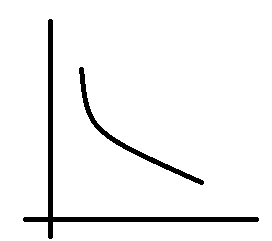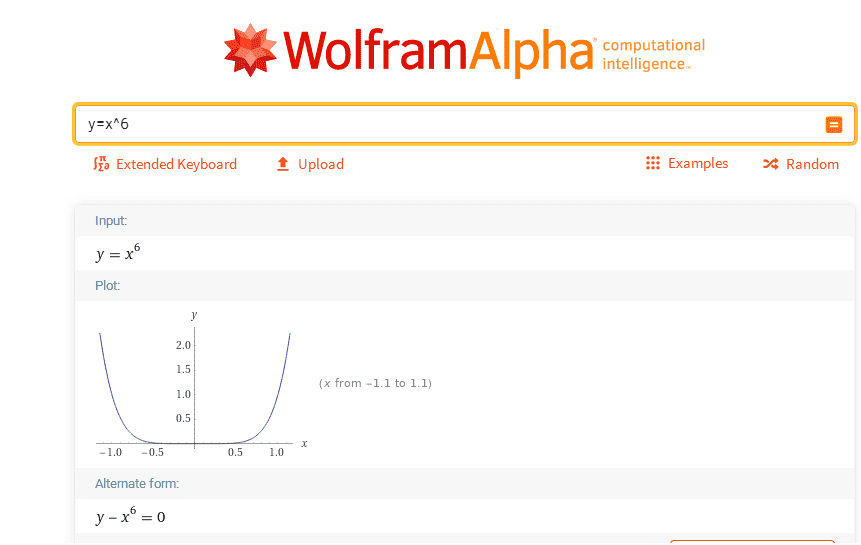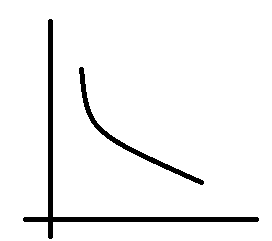# Complicated equation and Simple equation for the Same Curve?

pairofstrings
Summary
Complicated and simple representation of same curve.If I draw some arbitrary curve then that curve can be represented convolutedly in mathematical elements and it can also be represented in simple mathematical elements?

Thanks!

## Answers and Replies

Mentor
Summary:: Complicated and simple representation of same curve.

View attachment 280855If I draw some arbitrary curve then that curve can be represented convolutedly in mathematical elements and it can also be represented in simple mathematical elements?

Thanks!
Um, could you maybe define your terms more precisely, and give some examples (using LaTeX) for what you mean?

Gold Member
It really depends on how closely you want the equation to fit the curve. A gross approximation to the curve you drew is given by 1/x. You can get an early guess because that curve does not have a lot of "wiggles". If that is not good enough, there are some very sophisticated methods (called "interpolation methods") that will approximate the curve, going through a set of points exactly and smoothly.

pairofstringsI could write the equation of the above curve by beginning with first term as x^6 and with more terms I could inch closer and closer to the precise equation of the curve - this is what I am calling convoluted equation.

Mentor
View attachment 280867
I could write the equation of the above curve by beginning with first term as x^6 and with more terms I could inch closer and closer to the precise equation of the curve - this is what I am calling convoluted equation.
Um, no.pairofstrings
I could add, subtract, multiply, divide more terms after the first term to get the correct graph.

•berkeman
pairofstrings
Complicated:
x = 1-3+2-1+2

Simple:
x = 1

Gold Member
You can make high-order polynomials that go exactly through a set of points on the curve, but they tend to wiggle around a lot in between those points.

pairofstringsHow do I know if a graph is made out of complicated terms/many terms or simple terms?
Can the equation of the above curve be intricate?
Can the equation of the above curve be simple?

Can the equation for the above curve be intricated or simple?

Last edited:
Gold Member
Complicated:
x = 1-3+2-1+2

Simple:
x = 1
Your complicated and simple in this example are subjective.

pairofstrings
complicated and simple in this example are subjective
'x' can be a copy of a book.

pairofstrings
For the above curve, can the equation be both intricate, and simple? The equation might have been intricate but it was later simplified?

Mentor
View attachment 280870
How do I know if a graph is made out of complicated terms/many terms or simple terms?
Can the equation of the above curve be intricate?
Can the equation of the above curve be simple?

Can the equation for the above curve be intricated or simple?
Your Profile Page says you are working on your BS in math. What year are you at university?

Mentor
Of course imagine taking a basic trig identity subbing in other identities to make a very complex equation that you now know can be simplified.

##y = sec^2(x)= 1 + tan^2(x) ##

## = sin^2(x) + cos^2(x) + tan^2(x)##

Homework Helper
I can always make an equation more complicated. For instance ##y=x## can be rewritten as $$y=(x+1)(sin^2x+cos^2x) -1.$$ I cannot always make an expression "less complicated" without sacrificing arithmetic fealty. Your question really needs to be more specific

Last edited by a moderator:
Gold Member
'x' can be a copy of a book.
Okay, what' the sum of Newton's Principia and Averson's "An Introduction to C*-Algebras?" Is it greater than 6?

pairofstrings
Your Profile Page says you are working on your BS in math. What year are you at university?
I am self-studying. I haven't decided to go to a university. I am trying to find out what I will be doing in a university once I join it.

Complicated:
x = 1-3+2-1+2

Simple:
x = 1

I have one book then I lost three books then I acquired two more books then I lost one book then I acquired two more books.
Total books acquired: five.
Total books lost: four.
Total books in custody: one.

Okay, what' the sum of Newton's Principia and Averson's "An Introduction to C*-Algebras?" Is it greater than 6?

Why/how sum of names of two books be greater than 6?If I have a curve then can its equation be complex, and also simple?
The equation of the curve can become complicated anytime?
Can this curve have complicated equation?

Last edited:
Gold Member
Why/how sum of names of two books be greater than 6?

Sorry, I was just trying to get you to think more clearly about your own statements. I haven't had much success. You said 'x' , an integer in the example you provided, could be the copy of a book. My comment is intended to bring out the absurdity of your statement. I realize you're just waving your hands trying to express different levels of complexity. This is not the same as providing a working definition.

So, consider, is 3 more complicated, less complicated, or equally complicated than 7? One could define a concept of "complicated" for integers in any number of ways. We could use the number of prime factors, size, or some combination of these. To me, 4 - 2 is the same as 2 but you seem to think the extra step of reducing to 2 makes 4 - 2 more complicated. In terms of integers, your question is we could always reduce our expressions or choose not to. Uh, okay. So what?

•Mark44
pairofstrings
In terms of integers, your question is we could always reduce our expressions or choose not to. Uh, okay. So what?

Complicated:
x = 1-3+2-1+2

Simple:
x = 1

If I reduce the expression then I could know number of books in my custody.
If I don't reduce the expression then I could know what happened to the books over time.A gross approximation to the curve you drew is given by 1/x.
As FactChecker points out the curve can be as simple as y = 1/x but what I am trying to know is if this curve can have a complicated equation; something more than just y = 1/x and I would get the same curve.

Gold Member
If I reduce the expression then I could know number of books in my custody.
I don't know what you mean here. Do you mean that the point on the right is your current number of books?
If I don't reduce the expression then I could know what happened to the books over time.
So this is a plot of books in custody versus time?
View attachment 280920

As FactChecker points out the curve can be as simple as y = 1/x but what I am trying to know is if this curve can have a complicated equation; something more than just y = 1/x and I would get the same curve.
Sure. There are more complicated expressions that you might get to fit your curve better by adjusting parameters. The general shape of your curve (1/x) is a division by zero at x=0, so a better expression would probably need to have that aspect in it some way.

pairofstrings
I don't know what you mean here. Do you mean that the point on the right is your current number of books?

Complicated:
x = 1-3+2-1+2

Simple:
x = 1

If I solve x = 1 - 3 + 2 - 1 + 2 then I will get number of books in my custody.
If I don't solve x = 1 - 3 + 2 - 1 + 2 then I get information like number of books acquired, and number of books lost over time.

Gold Member
It sounds like you want something like ##B_{total} = B_1+B_2+B_3+B_4+...+B_n##

Mentor
Complicated:
x = 1-3+2-1+2

Simple:
x = 1
Your complicated and simple in this example are subjective.
'x' can be a copy of a book.
No.
From what you wrote later, x represents the number of books. It does not represent "a copy of a book." There is a big difference between how many things you are talking about, and the things themselves.

Your "complicated" equation above would not be considered complicated by anyone who has a grasp of very elementary arithmetic. If your intention is to get a degree in mathematics, you have a very long way to go.
I have one book then I lost three books then I acquired two more books then I lost one book then I acquired two more books.
Total books acquired: five.
Total books lost: four.
Total books in custody: one.
Again, no. This would necessarily be the net change in the number of books you have, not the total number of books you have. In other words, the 1 indicates that you now have one more book than you originally started with, which you didn't state. If you have only one book, you cannot possibly lose three books.

Gold Member
If I have a curve then can its equation be complex, and also simple?
yes.

Gold Member
I think the/a main issue here is that of parametrization of a curve up to equivalence ( change of coordinate). I think it is used often in algebraic geometry. Cc @mathwonk

Mentor
If I solve x = 1 - 3 + 2 - 1 + 2 then I will get number of books in my custody.
You are not "solving" this equation for x, since x is already isolated to one side. All you are doing is simplifying the not-very-complicated arithmetic expression on the right side.

pairofstrings
If you have only one book, you cannot possibly lose three books.
Thanks!
Is it correct if I replace "lose" with "owe" or "debt" in the statement I wrote?
How do I capture the notion of debt/owe/loss in a mathematical statement?
Sorry, I should have mentioned earlier that I have one book at the beginning.

Thanks!

Last edited:
Mentor
Is it correct if I replace "lose" with "owe" or "debt" in the statement I wrote?
How do I capture the notion of debt/owe/loss in a mathematical statement?
Sorry, I should have mentioned earlier that I have one book at the beginning.
Let's get rid of all three of these words: "owe", "debt", and "lose." Instead, imagine that you are running a shop that buys and sells books.

Let's also get rid of the equation you wrote -- x = 1 - 3 + 2 - 1 + 2 and focus just on the expression 1 - 3 + 2 - 1 + 2.

During one day, five customers come in. Let's assume that your shop starts the day with N books, where N is a reasonably large number.

Customer 1 sells 1 book.
Customer 2 buys 3 books.
Customer 3 sells 2 books.
Customer 4 buys 1 book.
Customer 5 sells 2 books.

At the end of the day, the number of books in the shop is N + 1 - 3 + 2 - 1 + 2, or more simply, N + 1 books. At the end of the day, the shop has 1 more book than at the start of the day.

pairofstrings
Can 'N' also be negative??

Mentor
Can 'N' also be negative??
Not in the scenario as I described it. To be meaningful, the seller could not sell more books at any time than there are on hand.

I'm just elaborating on the example you gave earlier.

pairofstrings
I can always make an equation more complicated. For instance y=x can be rewritten as y=(x+1)(sin^2x+cos^2x) -1.

Does y = x and y=(x+1)(sin^2x+cos^2x) -1 have the same meaning or is it the same graph but only the expressions are changing?

Last edited:
Mentor
Does y = x and y=(x+1)(sin^2x+cos^2x) -1 have the same meaning
Yes. The two equations are equivalent -- for a given value of x, both equations produce the same y value.
or is it the same graph but only the expressions are changing?
Yes to that, also. The graphs would be exactly the same. The only difference is that the expression on the right in the 2nd equation is unsimplified.

•pairofstrings
pairofstrings
The graphs would be exactly the same. The only difference is that the expression on the right in the 2nd equation is unsimplified.

I didn't think that I have online graphing calculators for producing graphs for these equations. Sorry about that.

Okay, so the unsimplified 2nd equation is destined to become 'x' on simplification? Like this: y = x? Is it possible to get anything else other than 'x' on simplification from this unsimplified 2nd equation?

Last edited:
Homework Helper
Yes. Making something "simpler" is more difficult: you reach the point where you cannot continue but you can never be certain. You can complexify ad infinitum

pairofstrings
The two equations are equivalent -- for a given value of x, both equations produce the same y value.

the unsimplified 2nd equation is destined to become 'x' on simplification? Like this: y = x? Is it possible to get anything else other than 'x' on simplification from this unsimplified 2nd equation?

Does y = x and y=(x+1)(sin^2x+cos^2x) -1 have the same context?
y = x may have context not similar to y = ( x + 1)( sin^2x + cos^2x ) - 1...

First one is linear equation (straight lines) and the second equation is trigonometric equation (side lengths and angles).

What could y = x be?
What could y=(x+1)(sin^2x+cos^2x) -1 be?

What does it mean if y is x and y is (x+1)(sin^2x+cos^2x) -1?

Thanks!

Last edited: